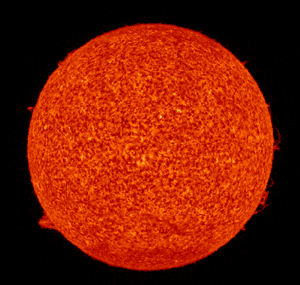PhysicalGeography.net | FUNDAMENTALS eBOOK

CHAPTER 6: Energy and Matter

# (g). The Solar Source of the Earth's Energy

Almost all of the energy that drives the various systems (climate systems, ecosystems, hydrologic systems, etc.) found on the Earth originates from the Sun. Solar energy is created at the core of the Sun when hydrogen atoms are fused into helium by nuclear fusion. For each second of this nuclear process, 700 million tons of hydrogen are converted into 695 million tons of helium. The remaining 5 million tons are turned into electromagnetic energy that radiates from the Sun's surface out into space.

The radiative surface of the Sun, or photosphere, has an average temperature of about 5800 Kelvins. Most of the electromagnetic radiation emitted from the Sun's surface lies in the visible band centered at 0.5 µm. The total quantity of energy emitted from the Sun's surface is approximately 63,000,000 Watts per square meter (W/m2 or Wm-2).Figure 6g-1: The Sun observed by SUMER instrument on the SOHO satellite on March 2-4, 1996. (Source: SOHO - SUMER Instrument).

The energy emitted by the Sun passes through space until it is intercepted by planets and other celestial objects. The intensity of solar radiation striking these objects is determined by a physical law known as the Inverse Square Law (see topic 6f). This law merely states that the intensity of the radiation emitted from the Sun varies with the squared distance from the source. As a result of this law, if the intensity of radiation at a given distance is one unit, at twice the distance the intensity will become only one-quarter. At three times the distance, the intensity will become only one-ninth of its original intensity at a distance of one unit, and so on.

Given the amount of energy radiated by the Sun and the average Earth-Sun distance of 149.5 million kilometers, the amount of radiation intercepted by the outer limits of the atmosphere can be calculated to be around 1370 W/m2. For general purposes, the energy output of the Sun can be considered constant. This of course is not entirely true. Scientists have shown that the output of the Sun is temporally variable. Some researchers have also suggested that the increase in the average global temperature over the last century may have been solar in origin. This statement, however, is difficult to prove because accurate data on solar output of radiation only goes back to about 1978 (see link).

Study Guide